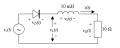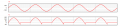# How do I graph the rectified voltage and voltage across inductor?

#### Wabbit

Joined Apr 17, 2017
2
I have a basic half wave rectifier with a supply source of 100 V rms. The circuit contains a 10 ohm resistor in series with a 10mH inductor.
I found the supply voltage to be 141 V. So graph is 141 peak to peak sinwave shape.
Now to find rectified voltage its basically 141 V positive peak with a small spike in the negative side which I do not know how to calculate.
Similarly I have the same problem with the inductor voltage.

#### crutschow

Joined Mar 14, 2008
24,963
I have a basic half wave rectifier with a supply source of 100 V rms. The circuit contains a 10 ohm resistor in series with a 10mH inductor.
So you only have a diode, a resistor and an inductor in the circuit?

What's the frequency of the signal?

•Wabbit

#### WBahn

Joined Mar 31, 2012
25,759
It always help to draw a schematic of the circuit and to label it with the various voltages and currents you are discussing. Otherwise we don't know where in the circuit you are talking about when you mention various waveforms.

If the supply voltage is 100 Vrms, then doesn't it go +141 V at the positive peak and then to -141 V for the negative peak? If so, how does that get reduced to only 141 Vpp (peak-to-peak)? Isn't it more like 282 Vpp?

What kind of solution are you looking for? Steady state, or with the startup transients?

What is the frequency of the input waveform? What is the time constant of the circuit?

•Wabbit

#### Wabbit

Joined Apr 17, 2017
2The frequency of the signal is 50 Hz.
I am told to sketch a graph of:
-the supply voltage Vs(t)
-rectified voltage vL(t)
-voltage across inductor vl(t)

So I managed to calculate Vs(t) = 100V * sqrt(2) = 141 V peak.
Obviously the the rectified voltage does not have any negative voltage and positive voltage should only occur every positive peaks align to the supply voltage. But there is a negative voltage spike due to the inductor which I do not know how to calculate. So the question would be how do I calculate the rectified voltage spike shown in VL?#### shteii01

Joined Feb 19, 2010
4,644
Where is the forward voltage of the diode?

#### MrAl

Joined Jun 17, 2014
7,591
Hello,

I was wondering that tooWhat is the forward voltage of the diode, can we consider it to be constant, can we consider it to be zero?

Also, what methods have you learned in the past for handling transient circuits that include an inductor?
This will help select the method for the solution.

What you have going for you in this circuit is that the inductor energy completely drains between cycles. What makes it more difficult (with a direct time analysis that is) is that the input is also time varying so it makes it more difficult to solve for the switching times of the diode. Because the inductor energy drains though that should make it easier because it will be the same for each cycle. In these circuits that also have a capacitor to store energy in the output it is sometimes much more difficult because the output also varies from cycle to cycle until steady state is reached, which can take 100 cycles or more.

LATER:
It turns out this is quite easy. To find out when the diode switches, look at the inductor current rather than the voltage. You can then figure out when to plot Vs to show VL because up to a certain point VL follows Vs, unless you have a diode drop and then VL will be less than Vs by that diode drop.
The only question then is why the diode voltage is shown as Vd(t). Does that mean we have to use a diode equation or just determine when it is on or off. If just on or off, then the above applies.

Last edited:

#### WBahn

Joined Mar 31, 2012
25,759
View attachment 124938
The frequency of the signal is 50 Hz.
I am told to sketch a graph of:
-the supply voltage Vs(t)
-rectified voltage vL(t)
-voltage across inductor vl(t)

So I managed to calculate Vs(t) = 100V * sqrt(2) = 141 V peak.
Obviously the the rectified voltage does not have any negative voltage and positive voltage should only occur every positive peaks align to the supply voltage. But there is a negative voltage spike due to the inductor which I do not know how to calculate. So the question would be how do I calculate the rectified voltage spike shown in VL?View attachment 124942
The voltage across the inductor is going to be whatever it needs to be in order to keep the current flow continuous. So focus on the current flow first.

#### MrAl

Joined Jun 17, 2014
7,591
The voltage across the inductor is going to be whatever it needs to be in order to keep the current flow continuous. So focus on the current flow first.
Hi,

The trick is that the inductor runs out of steam so the voltage no longer follows. That's the point of the solution...finding when it runs out of steam.

I found that this can be done in the frequency domain too, to keep it even simpler#### crutschow

Joined Mar 14, 2008
24,963
But there is a negative voltage spike due to the inductor which I do not know how to calculate
The negative spike occurs while the inductor is still carrying forward current after the input goes negative and the current is being supplied by the inductor.
The time for that is determined by the L/R time-constant of the circuit.